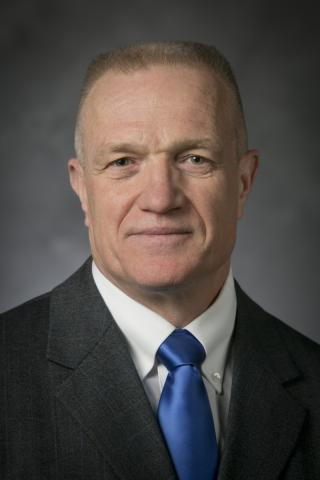# Robert Bryant

Duke University / UC Berkeley
Visit:
Monday, May 19, 2014 to Friday, May 23, 2014
Lectures:

Differential Systems and Holonomy

Abstract:  The concept of holonomy arose in the 19th century in the study of mechanical systems subject to `rolling constraints', such as an object rolling on a surface without slipping or twisting.  It quickly found application in differential geometry and mathematical physics, in the analysis of parallel translation associated to a connection and its relation with the curvature of the underlying geometric structures.  The subject continues to develop in a number of areas, including Riemannian, sub-Riemannian, and parabolic geometries, where questions of existence and uniqueness turn out to devolve on solving systems of differential equations that often do not fit into the standard types, but that can be approached using Cartan's theory of exterior differential systems.

In this series of lectures, I will introduce the ideas of the subject, illustrated by a series of examples drawn from mechanics and geometry, and discuss some of the interesting problems from differential equations that these examples raise as well as recent progress on their solutions.

Tuesday, May 20th - Lecture 1:  Rolling constraints and geometry

The problem of navigating in a space with rolling constraints arises in many contexts, from parking a car to robot hands manipulating small objects.  I will consider some examples and their analysis from the point of view of geometry, including an application of Cartan's famous 5-variables paper of 1910, which applies to the problem of one surface rolling over another without twisting or slipping and in which the first exceptional Lie group makes a surprising appearance.

Wednesday, May 21 - Lecture 2:  Prescribing holonomy groups via differential equations

While the appearance of the holonomy group in Riemannian geometry is well-known, the history contains some surprises, including Cartan's first nontrivial example in 1926, which is now recognized to be that of a Ricci-flat Kähler metric in complex dimension~2.  Many problems in geometry can be rephrased as the problem of locally prescribing a given group as holonomy, and this can be reduced to a PDE problem in a number of ways, but most of these lead to PDE that are either degenerate or overdetermined in some way, so the methods of exterior differential systems turn out to be essentially involved.  I will show how these problems can be expressed as existence problems for systems of Cartan structure equations and then analyzed using Cartan's generalization of Lie's third fundamental theorem.

Thursday May 22nd - Lecture 3:  Applications to the geometry of moduli spaces

One of the aspects of Penrose's twistor theory is expressing the solutions to certain systems of nonlinear PDE as geometries on moduli spaces of rational curves subject to some differential constraints, for example, Penrose's solution of the self-dual Einstein equations in terms of rational curves with a specified normal bundle in a three dimensional complex space.  In this final lecture, I will discuss a unified picture of a number of these classical differential geometry problems in which they can be expressed as prescribed holonomy problems and explain what the techniques of exterior differential systems has been able to say so far about them.Poster:Bryant.pdf